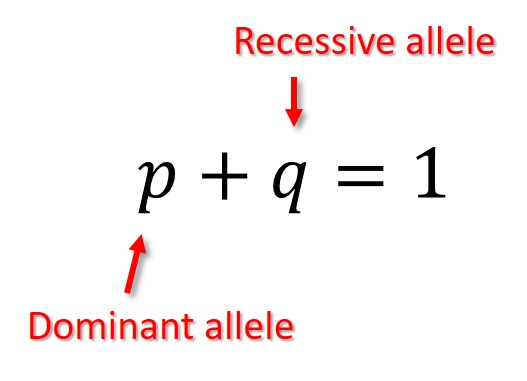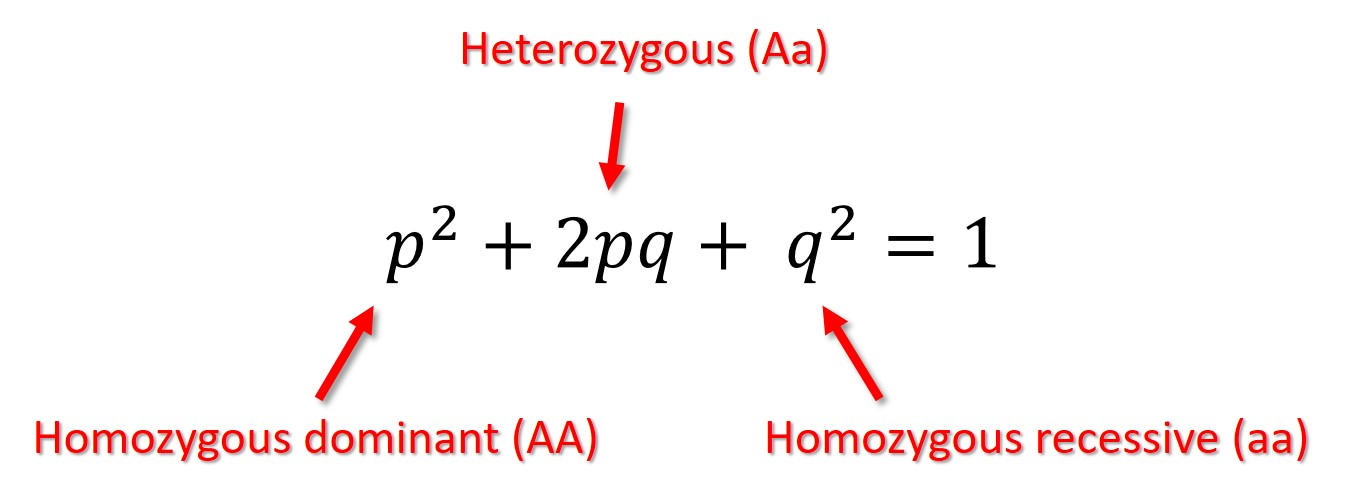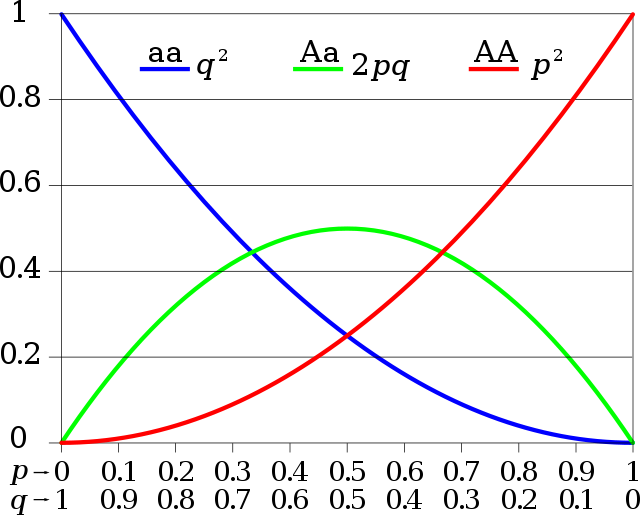Swati Talwar

# Hardy-Weinberg Equilibrium Study Guide

🗻 Big Picture: If a population is in Hardy-Weinberg equilibrium, the frequencies of alleles (gene versions) and genotypes (allele sets) in that population will remain constant throughout generations.

## Introduction

The statement of Hardy Weinberg law is: "In the absence of any evolutionary influences from generation to generation, the genotype and allele frequencies in a large, random-mating population remain constant." In other words, the rule states that if the frequency of distinct alleles in a population is already known, it is feasible to predict the anticipated frequencies of genotypes in a population under a restricted set of assumptions.

### Hardy-Weinberg Equations

The first Hardy-Weinberg equation estimates the frequency of certain alleles in a population.Source

The second Hardy-Weinberg equation is used to determine genotype frequencies.Source

The variable 'p2' denotes the homozygous dominant genotype (AA), '2pq' denotes the heterozygous genotype (Aa), and 'q2' denotes the homozygous recessive genotype (aa).

The following conclusions can be inferred through this law:

• Only sexual reproduction is possible.

• Mating is a random process.

• The population will continue to grow endlessly.

• The organisms are diploid, and the generations do not mix.

• In terms of sexes, allele frequencies are equal.

• No evidence of gene flow, selection, mutation, migration, or mixing could be found.

Differences between expected and actual results may arise if any assumptions are broken. The ramifications are completely dependent on the detour selected.

• Once a single generation of random mating is completed in a population, the law states that the population must have the Hardy Weinberg proportions (given genotypic frequencies).

• This population will not have the Hardy Weinberg proportions if the assumption of random mating is broken. Inbreeding is the most prevalent cause of non-random mating, and it causes the homozygosity of all genes to increase.

• If any of these four assumptions are broken, the population will still have the Hardy–Weinberg proportions at each generation, but the allele frequencies will shift over time. Changes in allele frequencies provide evidence for the occurrence of evolution in a population.

### Conditions Leading to Allele and Genotype Frequencies ChangesSource

Mutation: Mutation has just a little effect on allele frequencies. The mutation rate is in the range of 10-4 to 10-8, and this is the most common order of allele frequency changes. Recurrent mutations will conserve the alleles even if there is still a strong selection against them in the population.

Selection: Selection results in a fast shift in allele frequencies in most cases. Only a few forms of selections, such as balancing selection, can end in equilibrium with no loss of alleles. Still, others, such as directional selection, can eventually result in allele loss.

### Applications of Hardy-Weinberg Equilibrium

• The Hardy-Weinberg law is a mathematical criterion that may compare non-evolving populations to evolving populations.

• If allele frequencies are tracked throughout time, and anticipated frequencies are approximated using Hardy-Weinberg law values, then workings that drive population evolution may be theorized.

• The rule offers a blueprint for studying the population genetics of diploid species that fulfill the fundamental requirements of random mating, large populations, no mutation, migration, or selection.

## ✅ Conclusion

• The Hardy-Weinberg principle asserts that a population is indefinite and unaffected by sexual, natural selection, mutation, or migration in a particular population.

• The frequency of recessive genotypes may be used to compute the frequency of alleles, then calculate the square root of this frequency to get the recessive allele's frequency.

• The frequency of alleles in a population may be expressed as p + q = 1, where p is the dominant allele frequency, and q is the recessive allele frequency.

• The frequency of genotypes in a population may be calculated using the formula p2 + q2 + 2pq = 1, where p2 represents the frequency of homozygous dominant genotype, q2 represents the frequency of recessive genotype, and 2pq represents the frequency of heterozygous genotype.

### FAQs

1. What are the five conditions of the Hardy-Weinberg principle?

No mutation, no gene flow, a high population size, random mating, and no natural selection is required to preserve the Hardy-Weinberg equilibrium. Deviations from any of the Hardy-Weinberg equilibrium's five key underlying requirements can cause it to collapse.

2. What is the Hardy-Weinberg principle?

In the absence of disrupting events, the Hardy-Weinberg equilibrium states that genetic variation in a population will remain constant from generation to generation. Bringing new alleles into a population can upset the balance of allele frequencies.

3. What is meant by genetic equilibrium?

A variable version of a gene is known as an allele. Some genes exist in several distinct versions, all found on a chromosome at the same genetic locus. If there are two identical alleles at a locus, the genotype is homozygous, and if the two alleles differ, the genotype is heterozygous.

4. What are the 2 hardy Weinberg equations?

• p² + 2pq + q² = 1

• p + q = 1

5. What is the difference between allele and genotype frequency?

The relative frequency of an allele on a genetic locus in a population is measured by allele or gene frequency. The proportion of a specific genotype among all individuals in a community is known as genotypic frequency.

6. What does the Hardy-Weinberg model show?

The Hardy-Weinberg equation is a mathematical formula for calculating the genetic variation of an equilibrium population. The Hardy-Weinberg equation may be used to compute the frequencies of the three genotypes if the p and q allele frequencies are known.

7. What idea did Hardy and Weinberg disprove?

They rejected the theory that dominant allele percentages increase with time, causing recessive allele percentages to decrease.

8. What is the purpose of hardy Weinberg equilibrium?

Hardy-Weinberg Equilibrium (HWE) is a method for estimating the number of homozygous and heterozygous variant carriers in non-evolving populations based on allele frequency.

9. How do you know if Hardy-Weinberg is in equilibrium?

Scientists must observe at least two generations to determine if a population is in Hardy-Weinberg Equilibrium. The population is in Hardy-Weinberg Equilibrium if the allele frequencies are the same in both generations.

We hope you enjoyed studying this lesson and learned something cool about Hardy-Weinberg Equilibrium! Join our Discord community to get any questions you may have answered and to engage with other students just like you! Don't forget to download our App to experience our fun VR classrooms - we promise it makes studying much more fun! 😎

## Sources

1. Hardy-Weinberg equilibrium. https://www.nature.com/scitable/definition/hardy-weinberg-equilibrium-122/.

2. The Hardy-Weinberg Principle. https://www.nature.com/scitable/knowledge/library/the-hardy-weinberg-principle-13235724/.

3. Hardy-Weinberg Equilibrium in the Large Scale Genomic Sequencing Era. https://www.frontiersin.org/articles/10.3389/fgene.2020.00210/full.